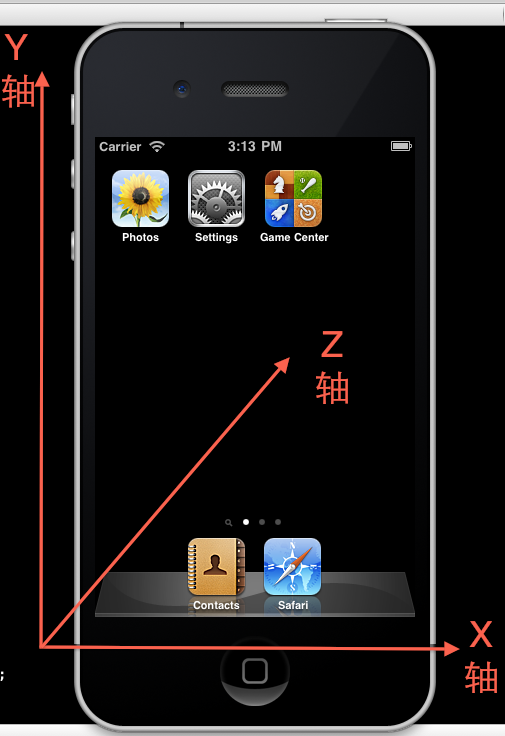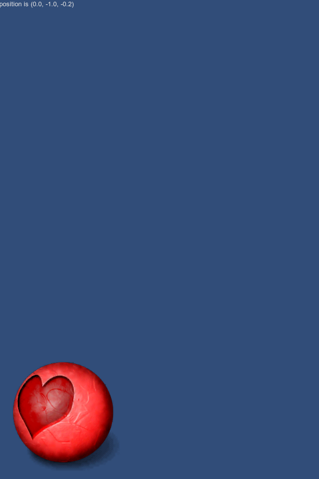Unity3D 游戏引擎之平面小球重力感应详解（十三）

Unity3D 游戏引擎之平面小球重力感应详解

手机重力感应应该对大多数开发者并不陌生，在新一代智能手机Android  IOS WP7 很多游戏都是使用手机自带重力感应功能制作的，强大的Unity3D 游戏引擎当然对这个也是完美支持的，今天由MOMO带大家学习3D 世界中的手机重力感应。本章我们的目标是实现一个小球在屏幕中通过摇晃手机重力加速度让小球在屏幕中移动。以前的Android系列开发文章中貌似也写过，其实原理都是一样一样一样的，废话不多说了。哇咔咔～～

X轴：home按键在下手机面朝天向右旋转90度重力分量为+1.0  向左旋转90度重力分量为-1.0

Y轴：home按键在上手机背朝自己重力分量为+1.0 home按键在下手机面朝自己重力分量为-1.0

Z轴：手机面朝地面重力分量为+1.0 手机面朝天空重力分量为-1.0

OK！ 有了这三组重要的数值我们就可以控制手机重力感应啦，紧接着我们看看小球重力感应的这个游戏小例子。Input.acceleration.x; 重力感应X轴的重力分量

Input.acceleration.y; 重力感应Y轴的重力分量

Input.acceleration.z; 重力感应Z轴的重力分量

new.js

```//小球的贴图
var round : Texture2D;

//小球在屏幕中显示的X Y坐标
var x = 0;
var y = 0;

//小球屏幕显示的最大 X Y 范围
var cross_x = 0;
var cross_y = 0;

function Start(){
//初始化赋值
cross_x = Screen.width -  round.width;
cross_y = Screen.height -  round.height;
}

function OnGUI () {

//整体显示 x y z 重力感应的重力分量
GUI.Label(Rect(0,0,480,100),"position is " + Input.acceleration);

//绘制小球
GUI.DrawTexture(Rect(x,y,256,256),round);
}

function Update(){

//根据重力分量修改小球的位置这里乘以30的意思是让小球移动的快一些
x += Input.acceleration.x * 30;
y += -Input.acceleration.y * 30;

//避免小球超出屏幕
if(x < 0){
x = 0;
}else if(x > cross_x){
x = cross_x;
}

if(y < 0){
y = 0;
}else if(y > cross_y){
y = cross_y;
}
}```